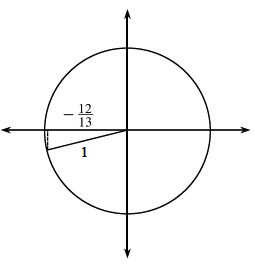### Home > CCA2 > Chapter 7 > Lesson 7.1.7 > Problem7-110

7-110.For angle $θ$ in the third quadrant, $\cosθ=−\frac{12}{13}$. Use this information to find each of the following values without using calculator.

Draw a unit circle and label the triangle for the given angle.Find the missing side of the triangle using the Pythagorean Theorem.
Use the missing side to evaluate the given trig ratios.
Remember that both $x\text{ and}\ y$ are negative in the third quadrant.

1. $\sin θ$

1. $\tan θ$

$\tan\theta=\frac{5}{12}$